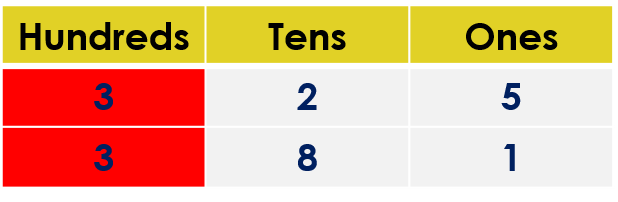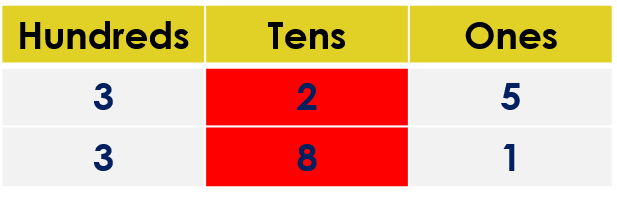1
visibility
Fill in the blank space with the correct sign. Use either <, > or =.
325  \$latex \framebox[0.5in]{}\$  381

The correct answer is "<".

It is easy!

• Compare the hundreds digits.
• If the hundreds digits are the same, then compare the tens digits.
• If tens digits are the same, then compare the ones digits.

Solution:

Observe that both numbers have three digits.

325 ____ 381

Comparing the hundreds digits:We observe that both digits are the same.

Comparing the tens digits:We observe that both digits are NOT the same.

• The tens digit in the number 325 is 2.
• The tens digit in the number 381 is 8.

But, 2 is less than 8.

So, 325 is less than 381.

That is, 325 < 381.